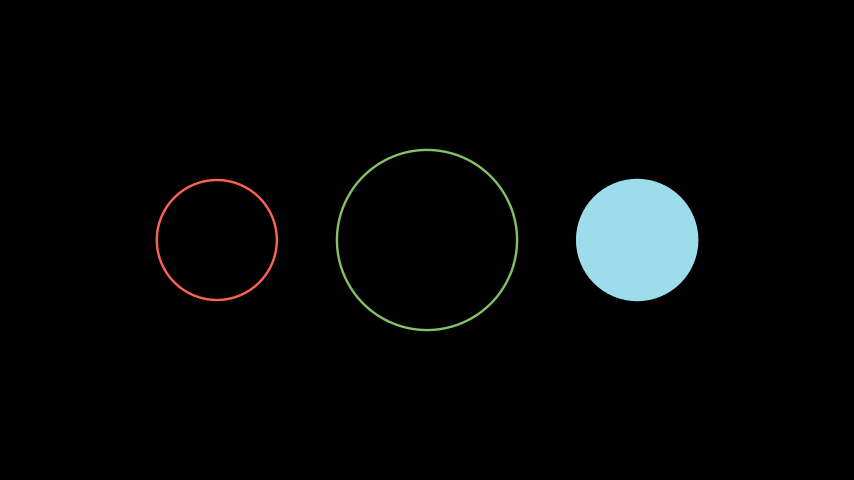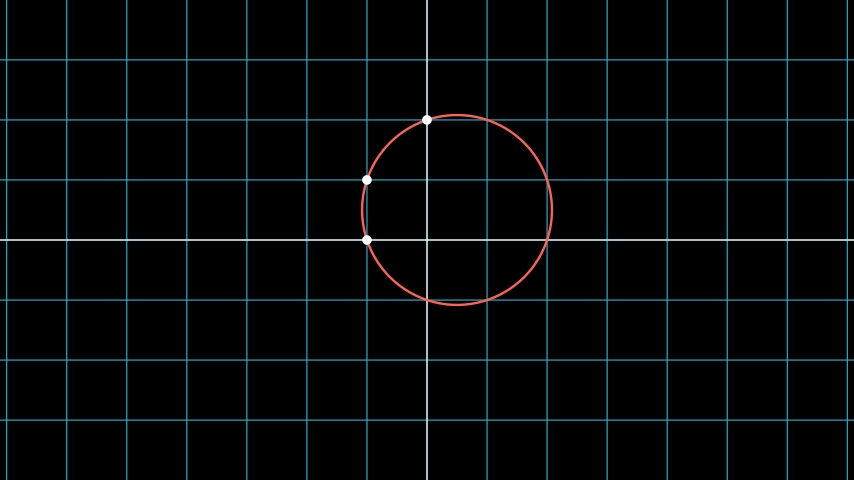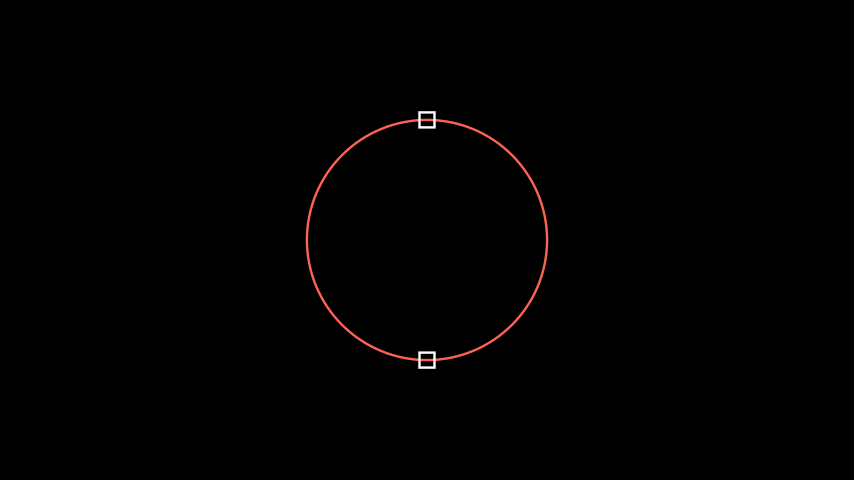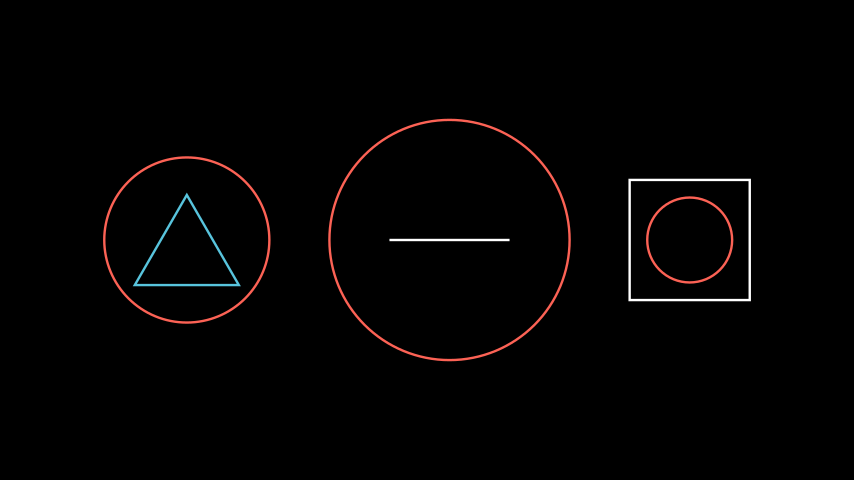# Circle#

Qualified name: `manim.mobject.geometry.arc.Circle`

Bases: `Arc`

A circle.

Parameters:
• color (ParsableManimColor) – The color of the shape.

• kwargs – Additional arguments to be passed to `Arc`

• radius (float | None) –

Examples

Example: CircleExample```from manim import *

class CircleExample(Scene):
def construct(self):

circle_group = Group(circle_1, circle_2, circle_3).arrange(buff=1)
```
```class CircleExample(Scene):
def construct(self):

circle_group = Group(circle_1, circle_2, circle_3).arrange(buff=1)

```

Methods

 `from_three_points` Returns a circle passing through the specified three points. `point_at_angle` Returns the position of a point on the circle. `surround` Modifies a circle so that it surrounds a given mobject.

Attributes

 `animate` Used to animate the application of any method of `self`. `animation_overrides` `color` `depth` The depth of the mobject. `fill_color` If there are multiple colors (for gradient) this returns the first one `height` The height of the mobject. `n_points_per_curve` `sheen_factor` `stroke_color` `width` The width of the mobject.

Initialize self. See help(type(self)) for accurate signature.

Parameters:
• radius (float | None) –

• color (Union[ManimColor, int, str, Tuple[int, int, int], Tuple[float, float, float], Tuple[int, int, int, int], Tuple[float, float, float, float], ndarray[Any, dtype[int64]], ndarray[Any, dtype[float64]]]) –

Return type:

None

static from_three_points(p1, p2, p3, **kwargs)[source]#

Returns a circle passing through the specified three points.

Example

Example: CircleFromPointsExample```from manim import *

class CircleFromPointsExample(Scene):
def construct(self):
circle = Circle.from_three_points(LEFT, LEFT + UP, UP * 2, color=RED)
dots = VGroup(
Dot(LEFT),
Dot(LEFT + UP),
Dot(UP * 2),
)
```
```class CircleFromPointsExample(Scene):
def construct(self):
circle = Circle.from_three_points(LEFT, LEFT + UP, UP * 2, color=RED)
dots = VGroup(
Dot(LEFT),
Dot(LEFT + UP),
Dot(UP * 2),
)

```
Parameters:
• p1 (Point3D) –

• p2 (Point3D) –

• p3 (Point3D) –

Return type:

Self

point_at_angle(angle)[source]#

Returns the position of a point on the circle.

Parameters:

angle (float) – The angle of the point along the circle in radians.

Returns:

The location of the point along the circle’s circumference.

Return type:

`numpy.ndarray`

Examples

Example: PointAtAngleExample```from manim import *

class PointAtAngleExample(Scene):
def construct(self):
p1 = circle.point_at_angle(PI/2)
p2 = circle.point_at_angle(270*DEGREES)

s1 = Square(side_length=0.25).move_to(p1)
s2 = Square(side_length=0.25).move_to(p2)
```
```class PointAtAngleExample(Scene):
def construct(self):
p1 = circle.point_at_angle(PI/2)
p2 = circle.point_at_angle(270*DEGREES)

s1 = Square(side_length=0.25).move_to(p1)
s2 = Square(side_length=0.25).move_to(p2)

```
surround(mobject, dim_to_match=0, stretch=False, buffer_factor=1.2)[source]#

Modifies a circle so that it surrounds a given mobject.

Parameters:
• mobject (Mobject) – The mobject that the circle will be surrounding.

• dim_to_match (int) –

• buffer_factor (float) – Scales the circle with respect to the mobject. A buffer_factor < 1 makes the circle smaller than the mobject.

• stretch (bool) – Stretches the circle to fit more tightly around the mobject. Note: Does not work with `Line`

Return type:

Self

Examples

Example: CircleSurround```from manim import *

class CircleSurround(Scene):
def construct(self):
triangle1 = Triangle()
circle1 = Circle().surround(triangle1)
group1 = Group(triangle1,circle1) # treat the two mobjects as one

line2 = Line()
circle2 = Circle().surround(line2, buffer_factor=2.0)
group2 = Group(line2,circle2)

# buffer_factor < 1, so the circle is smaller than the square
square3 = Square()
circle3 = Circle().surround(square3, buffer_factor=0.5)
group3 = Group(square3, circle3)

group = Group(group1, group2, group3).arrange(buff=1)
```
```class CircleSurround(Scene):
def construct(self):
triangle1 = Triangle()
circle1 = Circle().surround(triangle1)
group1 = Group(triangle1,circle1) # treat the two mobjects as one

line2 = Line()
circle2 = Circle().surround(line2, buffer_factor=2.0)
group2 = Group(line2,circle2)

# buffer_factor < 1, so the circle is smaller than the square
square3 = Square()
circle3 = Circle().surround(square3, buffer_factor=0.5)
group3 = Group(square3, circle3)

group = Group(group1, group2, group3).arrange(buff=1)